# torch.nn 和 torch.functional 的区别

Pytorch打怪路（一）pytorch进行CIFAR-10分类（2）定义卷积神经网络Pytorch打怪路（一）pytorch进行CIFAR-10分类（3）定义损失函数和优化器

# 2.代码

[python] view plain
?
1. # 首先是调用Variable、 torch.nn、torch.nn.functional
2. from torch.autograd import Variable   # 这一步还没有显式用到variable，但是现在写在这里也没问题，后面会用到
3. import torch.nn as nn
4. import torch.nn.functional as F
5.
6.
7. class Net(nn.Module):                 # 我们定义网络时一般是继承的torch.nn.Module创建新的子类
8.     def __init__(self):
9.         super(Net, self).__init__()   # 第二、三行都是python类继承的基本操作,此写法应该是python2.7的继承格式,但python3里写这个好像也可以
10.         self.conv1 = nn.Conv2d(365)       # 添加第一个卷积层,调用了nn里面的Conv2d（）
11.         self.pool = nn.MaxPool2d(22)        # 最大池化层
12.         self.conv2 = nn.Conv2d(6165)      # 同样是卷积层
13.         self.fc1 = nn.Linear(16 * 5 * 5120# 接着三个全连接层
14.         self.fc2 = nn.Linear(12084)
15.         self.fc3 = nn.Linear(8410)
16.
17.     def forward(self, x):                  # 这里定义前向传播的方法，为什么没有定义反向传播的方法呢？这其实就涉及到torch.autograd模块了，
19.         x = self.pool(F.relu(self.conv1(x)))  # F是torch.nn.functional的别名，这里调用了relu函数 F.relu()
20.         x = self.pool(F.relu(self.conv2(x)))
21.         x = x.view(-116 * 5 * 5)  # .view( )是一个tensor的方法，使得tensor改变size但是元素的总数是不变的。
22.                                     #  第一个参数-1是说这个参数由另一个参数确定， 比如矩阵在元素总数一定的情况下，确定列数就能确定行数。
23.                                     #  那么为什么这里只关心列数不关心行数呢，因为马上就要进入全连接层了，而全连接层说白了就是矩阵乘法，
24.                                     #  你会发现第一个全连接层的首参数是16*5*5，所以要保证能够相乘，在矩阵乘法之前就要把x调到正确的size
25.                                     # 更多的Tensor方法参考Tensor: http://pytorch.org/docs/0.3.0/tensors.html
26.         x = F.relu(self.fc1(x))
27.         x = F.relu(self.fc2(x))
28.         x = self.fc3(x)
29.         return x
30.
31.  # 和python中一样，类定义完之后实例化就很简单了，我们这里就实例化了一个net
32. net = Net()
# 首先是调用Variable、 torch.nn、torch.nn.functional
from torch.autograd import Variable   # 这一步还没有显式用到variable，但是现在写在这里也没问题，后面会用到
import torch.nn as nn
import torch.nn.functional as F

class Net(nn.Module):                 # 我们定义网络时一般是继承的torch.nn.Module创建新的子类
def __init__(self):
super(Net, self).__init__()   # 第二、三行都是python类继承的基本操作,此写法应该是python2.7的继承格式,但python3里写这个好像也可以
self.conv1 = nn.Conv2d(3, 6, 5)       # 添加第一个卷积层,调用了nn里面的Conv2d（）
self.pool = nn.MaxPool2d(2, 2)        # 最大池化层
self.conv2 = nn.Conv2d(6, 16, 5)      # 同样是卷积层
self.fc1 = nn.Linear(16 * 5 * 5, 120) # 接着三个全连接层
self.fc2 = nn.Linear(120, 84)
self.fc3 = nn.Linear(84, 10)

x = self.pool(F.relu(self.conv1(x)))  # F是torch.nn.functional的别名，这里调用了relu函数 F.relu()
x = self.pool(F.relu(self.conv2(x)))
x = x.view(-1, 16 * 5 * 5)  # .view( )是一个tensor的方法，使得tensor改变size但是元素的总数是不变的。
#  第一个参数-1是说这个参数由另一个参数确定， 比如矩阵在元素总数一定的情况下，确定列数就能确定行数。
#  那么为什么这里只关心列数不关心行数呢，因为马上就要进入全连接层了，而全连接层说白了就是矩阵乘法，
#  你会发现第一个全连接层的首参数是16*5*5，所以要保证能够相乘，在矩阵乘法之前就要把x调到正确的size
# 更多的Tensor方法参考Tensor: http://pytorch.org/docs/0.3.0/tensors.html
x = F.relu(self.fc1(x))
x = F.relu(self.fc2(x))
x = self.fc3(x)
return x

# 和python中一样，类定义完之后实例化就很简单了，我们这里就实例化了一个net
net = Net()



# 3.涉及知识点

## ①神经网络工具箱 torch.nn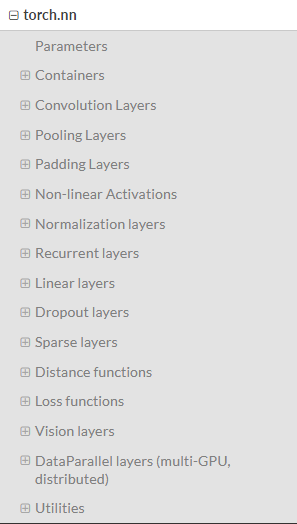### a.  Container中的Module，也即nn.Module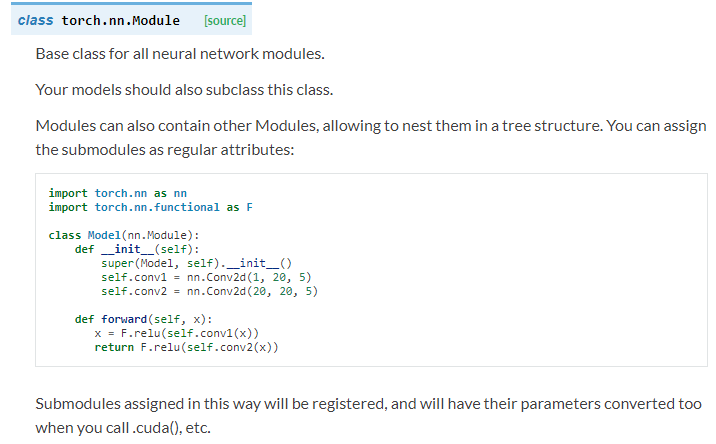### b.  convolution layers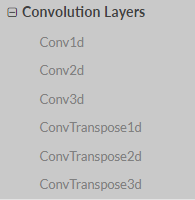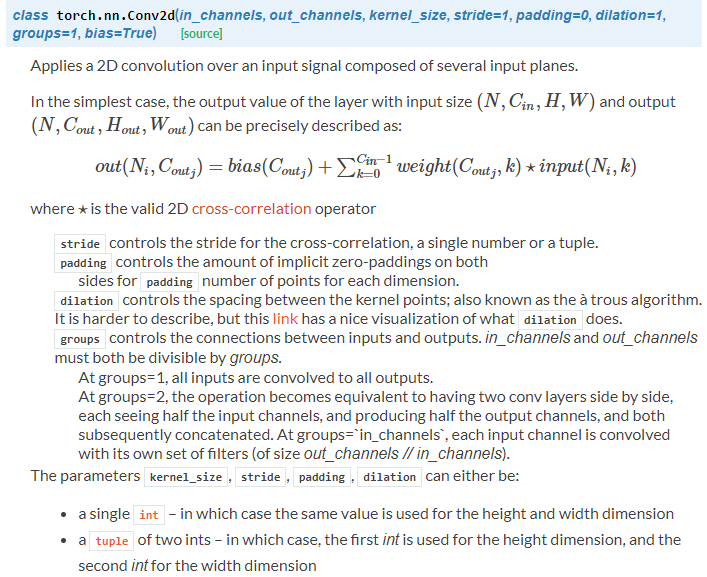### c.  pooling layers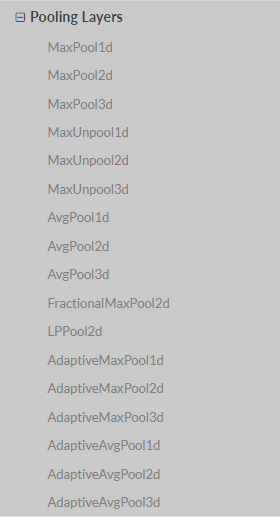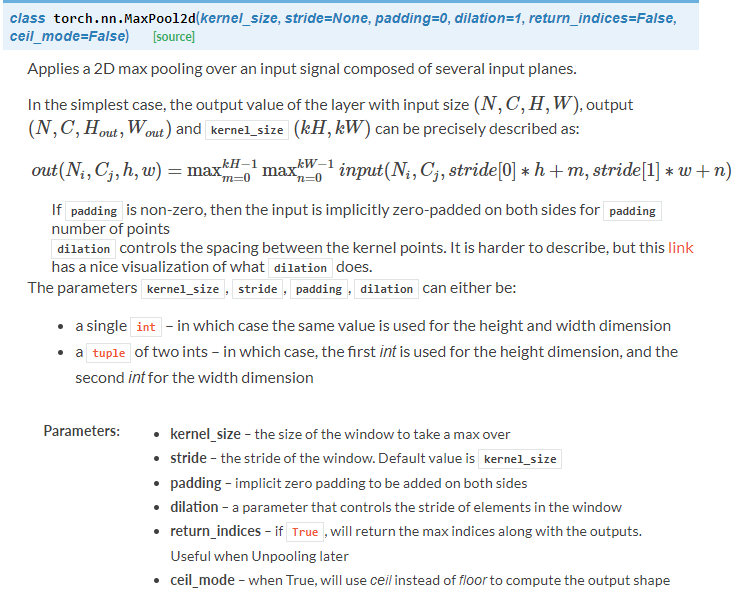### d.  Linear layer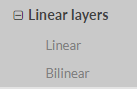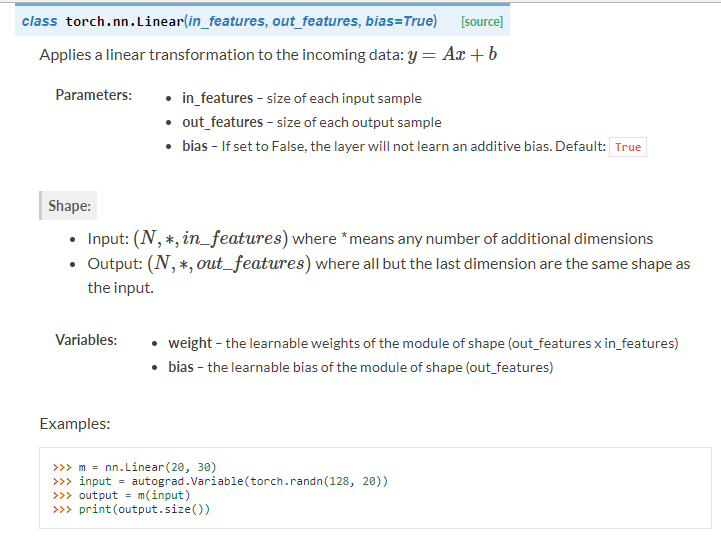### e.   Non-linear Activations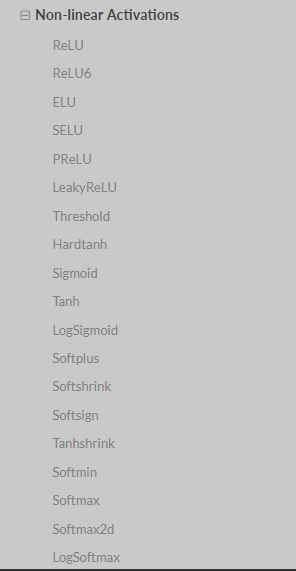## ②torch.nn.functional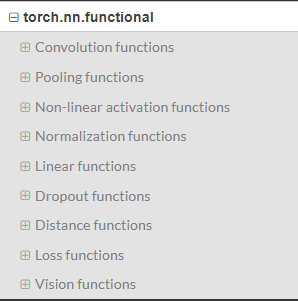torch.nn中大多数layer在torch.nn.funtional中都有一个与之对应的函数。二者的区别在于：
torch.nn.Module中实现layer的都是一个特殊的类，可以去查阅，他们都是以class xxxx来定义的，会自动提取可学习的参数
nn.functional中的函数，更像是纯函数，由def function( )定义，只是进行简单的数学运算而已。

        </div>
</div>©️2019 CSDN 皮肤主题: 大白 设计师: CSDN官方博客